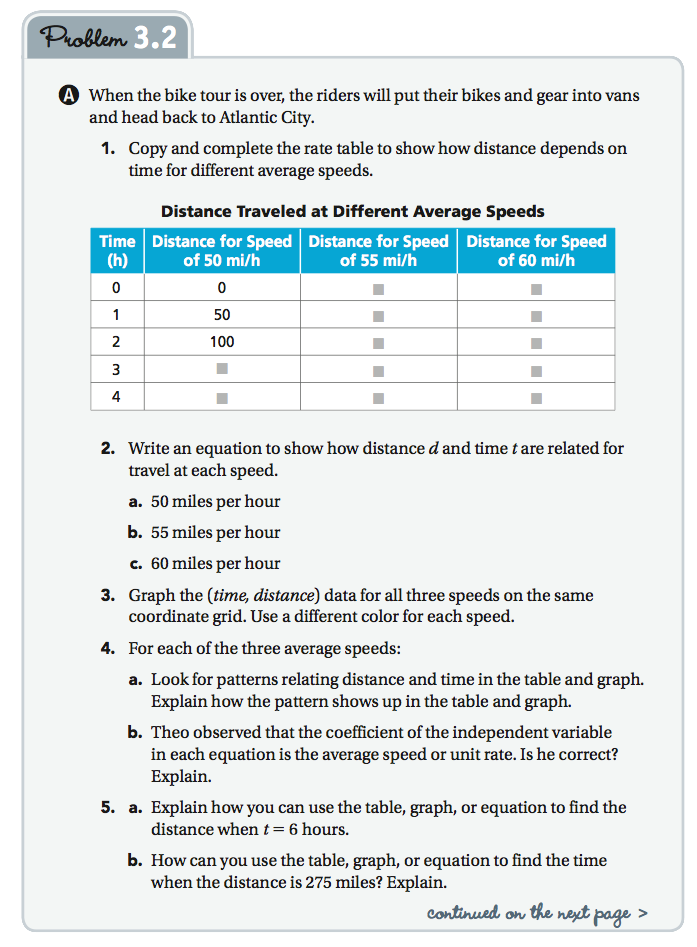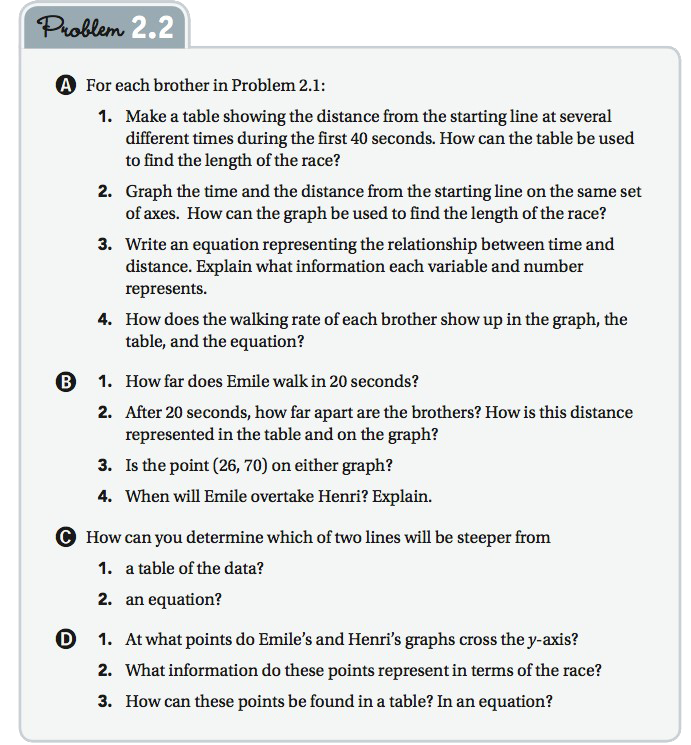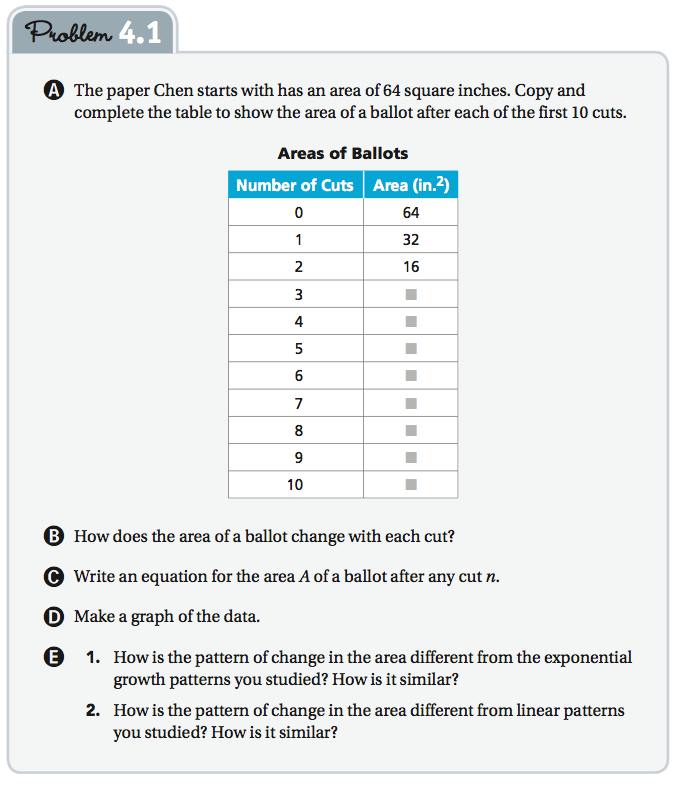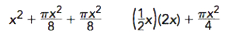Display Accessibility Tools

# Algebra and Functions

CMP is a problem-centered curriculum in which quantities or variables naturally arise in the context of a problem. In this curriculum, it makes sense to think about how variables are related, how they can be represented, and the information we can get from the way they are represented. CMP’s approach intertwines the CCSSM algebra and functions strands into one coherent algebra and functions, strand across three years.

The nine CMP algebra and functions Units challenge students, from the very first Investigation in sixth grade to the very last Investigation in eighth grade, to represent algebraic relationships in words, tables, graphs, and symbols. Writing and reasoning with symbolic expressions, an important goal of the CCSSM, is incorporated throughout the program. Equations are first understood as symbolic rules that relate independent and dependent variables. One-variable equations are, therefore, specific instances, or snapshots, of function relationships, in which one of the variables is a known quantity. A natural outcome of combining functions and algebra through problem situations is that solving one-variable equations is first done using graphs, tables, or numeric reasoning and then by symbolic methods. Manipulating symbols to write equivalent expressions, another important CCSSM goal, reveals new insights into the relationship that represents a problem situation, and opens up other solution strategies.

As students continue to develop their understanding of linear and non linear, they also develop deeper understandings of expressions and equations that are required to represent more complicated situations and vice versa more complicated situations push the understanding of linear relationships. Because of CMP’s emphasis on reasoning about and with relationships during grades 6–8, students are well prepared for the Common Core Functions standards.

## Note

A majority of the algebra occurs in the 8th grade units. By completing all eight units in 8th grade, students will have completed the CCSS requirements for 8th Grade and Algebra I. By completing parts of six or seven units in 8th grade, students will have completed the requirements for CCSSM 8th Grade. For more information see: Math Content by Unit.

## Understanding Variables

Students enter sixth grade with some understanding of operations, such as when to apply them and how they are related, skill in writing number sentences to represent problem situations, and the ability to find an unknown in a number sentence. They have had opportunities to analyze patterns. CMP builds on these early understandings of the meaning of operations and the uses of variables.

From the perspective of developing an algebra and functions strand, variables are not simply letters that stand for unknown quantities, and operations are not simply instructions to compute a numerical result, although these are important parts of the picture. Rather, variables are quantitative attributes of objects, patterns, or situations, which change in response to changes in other quantities; and operations are the building blocks of functional relationships.

## Goals

The overarching goals of the Algebra and Functions strand are:

• Developing understanding of variables and relationships among variables
• Recognizing, interpreting, and representing relationships, including linear, quadratic, exponential, inverse variation, and polynomial functions
• Writing, interpreting, and using equivalent expressions and equations
• Solving equations and inequalities, including systems of equations

There are nine Units in the Algebra and Functions strand, over which these goals are developed as students refine their understanding of algebraic concepts. The first Algebra Unit is Variables and Patterns, in the sixth grade. This is not students' first encounter with variables, however. They have seen variable expressions, equations, patterns, and relationships in earlier, non algebra-strand Units. In Covering and Surrounding, students develop formulas and procedures, stated in words and symbols, for finding areas of figures. In Let's Be Rational and Decimal Ops, students write number sentences to solve problems and use fact-family knowledge to rewrite and solve equations.

## Developing Understanding of Variables and Relationships Among Variables

In Variables and Patterns, students look for patterns in graphs, tables, and equations representing relationships between variables, for various situations. For example,in Problem 3.2, part of which is shown below, students observe that all the graphs are straight lines, all the equations are in the form y = mx, and the point (1, m) is on every graph. They also make the connection that the unit rate is m miles per 1 hour.As seen in Problem 3.2, the primary focus of Variables and Patterns is relationships among variables. Students are also working with these representations and solving equations. This is the beginning of the development of other goals in the algebra and functions strand: recognizing linear relationships, writing equations, and solving equations. In the seventh-grade Unit Moving Straight Ahead, students learn to use properties of equality to solve one-variable linear equations.

## Recognizing, Interpreting, and Representing Functional Relationships

In Moving Straight Ahead, the Algebra and Functions strand Unit in Grade 7, students identify the characteristics of a linear relationship represented in a table, graph, or equation. The main goal is to have students recognize the constant rate of change in the dependent variable as the independent variable changes by constant increments. Students also learn to solve one-variable linear equations.

In Problem 2.1 of Moving Straight Ahead, students decide how long a walking race should be between two brothers who walk at different rates and start at different points, if they want the little brother to win. To be sure that multiple representations for Problem 2.1 are compared and discussed, Problem 2.2 follows up by asking for a table, a graph, and equations to represent the situation. In this way, students begin to understand constant rate of change and the y-intercept, and how these appear in a table, graph, and equation.

As seen in Problem 2.2 (below), a major focus in Moving Straight Ahead is recognizing the characteristics of a linear function in its various representations. Developing an understanding of relationships among variables also continues to be a focus throughout. Later in the Unit, slope and y-intercept are formally defined.Another focus is on solving equations such as 45 + n = 2.5n. Each expression, 45 +  = 2.5n, represents the distance each brother has walked. Students learn to use the properties of equality to solve linear equations. In the Grade 8 Unit It’s in the System, students develop various strategies for solving systems of equations.For now, they might use numeric reasoning, a table, or a graph to solve the system y = 45 + n and y = 2.5n, or set the two expressions for y equal and solve for n.

The Algebra and Functions strand dominates in Grade 8 with six Units of focus. In Thinking With Mathematical Models, students investigate the nonlinear pattern of inverse variation. In Growing, Growing, Growing, students write equations for exponential functions and learn to recognize the patterns of exponential growth or decay from tables, graphs, and equations. These experiences with different relationships give students opportunities to compare linear and nonlinear patterns and to compare nonlinear patterns with each other. For example, as they work on Growing, Growing, Growing, Problem 4.1 (shown below), some students make the conjecture that the relation in the Problem is like an inverse variation situation they have seen earlier.In both Thinking With Mathematical Models and Growing, Growing, Growing, as seen above, one major goal is to represent, compare, and contrast linear relationships with inverse variation and exponential relationships. In Frogs, Fleas, and Painted Cubes and in Function Junction, students continue to represent and interpret nonlinear relationships, including quadratic and polynomial relationships. In Function Junction, functional notation is formally introduced and used to represent functions symbolically and to study new functions such as piece wise linear, absolute value, and polynomial functions.

### Writing, Interpreting, and Using Equivalent Expressions

Students’ first experiences with equivalence are with fractions and ratios, in Comparing Bits and Pieces (Grade 6). Their first experience with equivalence of symbolic expressions and equations comes in Variables and Patterns (Grade 6), as they write an expression for the number of beams needed to make a tower in Problem 4.1 and find many possible expressions, such as B = 4 + 3(n - 1) and B = 1 + 3n.

To justify the equivalence of these expressions for B, students should be able to point out that substituting a value for n in either equation gives the same value for B and that using the Distributive Property on the first equation transforms it into the second equation.

Students also validate the reasoning behind each expression and come up with these different expressions and explanations:

• B = 4 + 3(- 1)
The first frame needs 4 beams, but each frame after that needs only 3.
• B = 1 + 3n
You put down 1 beam and then keep adding 3 beams to make every step.

The primary focus in Variables and Patterns is on understanding relationships among variables. As students explain their reasoning, they naturally begin to interpret and use equivalent expressions. While the focus in Variables and Patterns is on a general introduction to relationships and ways to represent these relationships, the characteristics of linear relationships also begin to emerge.

In Frogs, Fleas, and Painted Cubes (Grade 8), students encounter situations that can be modeled with quadratic functions. They recognize that different, equivalent expressions may give useful information about the related graphs. For example, in Problem 2.3, students write two expressions for the area of the rectangle in red.

In Frogs, Fleas, and Painted Cubes (Grade 8), students encounter situations that can be modeled with quadratic functions. They recognize that different, equivalent expressions may give useful information about the related graphs. For example, in Problem 2.3 (shown to the right), students write two expressions for the area of the rectangle in red.

Writing and interpreting equivalent expressions continues to be a major focus in Say It With Symbols. For example, in Problem 1.3, students examine the structure of a given expression and write equivalent expressions around the topic of the area of parts of a pool. For example, the two expressions below that could be written are equivalent.The symbols are attached to context and thus relay different aspects of information about the situation. Many other equivalent expressions are possible.

In addition to working with equivalent linear and quadratic expressions, students work with expressions in which the variable is raised to powers greater than 2. The rules for exponents are developed in Growing, Growing, Growing.

### Solving Equations and Inequalities

Students come to middle school knowing a bit about number sentences and finding unknown quantities. They use informal methods, such as fact families, to solve problems in Grade 6, particularly in Let’s Be Rational and Decimal Ops. In Variables and Patterns, the first algebra Unit, students continue to use these informal methods, but, since they see two-variable relationships, they can also use graphs and tables to find solutions. In Moving Straight Ahead (Grade 7), properties of equality are introduced. From that point on, students are familiar with graphical, tabular, and symbolic ways to solve linear equations.

Moving Straight Ahead introduces symbolic methods of solving equations using coins and pouches. Each pouch holds the same but variable numbers of coins. For example, 3x + 4 represents three pouches and four coins. In It’s in the System (Grade 8), students recall that method of representation and use it to represent systems of linear equations.

It’s in the System pulls together various methods for solving systems of equations and connects these to students’ prior experiences, particularly in Moving Straight Ahead. CMP students often attach conceptual ideas to the problem context in which they were first introduced.

Students solve quadratic equations in Frogs, Fleas, and Painted Cubes, using factoring and the principle that if pq = 0, than either p or q equals 0. Of course, not every quadratic expression can be factored, so another equivalent form for a quadratic expression is investigated in Function Junction. Students learn how to write the equation of any quadratic function in the equivalent vertex form. Once students have established this strategy, they can use it to solve any quadratic equation, thus completing the story about solving quadratic equations. They can also appreciate the derivation of the quadratic formula and see rich connections between graphical solutions and symbolic solutions.

Lastly, inequalities are addressed in several units beginning with Variables and Patterns in grade 6. In grade 7, in Moving Straight Ahead, Problem 2.3, students are given the equations for two different plans for buying T-shirts: Cn = 4.5n and Cm = 49 + n. They use graphs and tables to find when the costs of the two different plans are equal. Later, they use symbolic methods for finding the number of T-shirts for which the two plans are equal. This leads to the question, “For what number(s) of T-shirts is plan Cm less than plan Cn?” That is, when is Cm <  Cn? Students use their knowledge of when the two plans are equal to answer the questions about inequalities. Solving inequalities, including symbolic methods, continues in Say It With Symbols and again in It’s in the System.

## Conclusion

In the first CMP Algebra and Functions Unit, Variables and Patterns, students represent relationships with graphs, tables, and equations. They are thus introduced to patterns of change, a major organizing principle for the Algebra and Functions strand. As students work their way through subsequent algebra Units, they see and solve increasingly complex problems. They use multiple representations of and investigate different types of relationships, including linear, inverse, quadratic, exponential, polynomial, piece wise, and absolute-value relationships. As students develop understanding of these relationships and use them to model new situations, they also grow in their sophistication to interpret and write equivalent expressions and equations, and to solve equations and systems of equations.

Equivalence is another important organizing principle of the Algebra and Functions strand. It plays a major role from the first Unit, Variables and Patterns, in which students use properties and operations of real numbers to write and interpret equivalent expressions, to the last Unit, Function Junction, in which students add, subtract, and multiply polynomials and rewrite the results in equivalent forms. Additionally, in Function Junction, students analyze the quadratic formula by comparing it to the steps needed to rewrite the equation of a quadratic function in an equivalent, vertex form.

Developing strategies for solving various types of equations is a major theme of the Algebra and Functions strand. In Variables and Patterns, students may solve equations by using a table or graph, or by using fact families. In Moving Straight Ahead, they use properties of equality to solve equations. Solving an equation with more than one variable for one of the variables extends this work and is used in It’s in the System and also in Function Junction. The CMP materials encourage students to make connections among symbolic and graphical representations and strategies for solving linear, quadratic, and polynomial equations. Simultaneously, they develop and refine their skills at symbol manipulation.